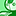Announcements
Applications are open for the 2024 Qlik Luminary Program. Apply by December 15 here.
cancel
Showing results for
Did you mean:Creator

## Rolling 4 quarters based on todays date

Hi

Am looking to create a table which has summed values outlining each of the last 4 quarters based on the current date.

In my script I have a Quarter field generated using the following:

Dual('Q' & Ceil(Month([SUBS_INVOICES_INVOICE_DATE])/3) & '-' & Year([SUBS_INVOICES_INVOICE_DATE]), QuarterStart([SUBS_INVOICES_INVOICE_DATE])) as SUBS_INVOICE_QUARTER

I wish to have a rolling table which has the following expression, I can get it working fine but it is hardcoded as you can see; it uses a variable of CQTR= Q1-2019. I need a set of variables which follows the same format as the below:

=Dual('Q' & Ceil(Month(Today())/3) & '-' & Year(Today()), QuarterStart(Today()))

The differing variables WILL ALSO GIVE ME THE QUARTER BEFORE AND BEFORE THAT AND BEFORE THAT. I envisage differing variables for each will drop into the following expression in the table.

=Sum( {\$<[SUBS_INVOICE_QUARTER] = {'\$(CQTR)'}>}SUBS_INVOICES_INVOICE_AMOUNT )

Thanks

Martin

1 Solution

Accepted SolutionsMVP

May be this

`=Dual('Q' & Ceil(Month(MonthStart(Today(), -3))/3) & '-' & Year(MonthStart(Today(), -3)), QuarterStart(MonthStart(Today(), -3)))`
`=Dual('Q' & Ceil(Month(MonthStart(Today(), -6))/3) & '-' & Year(MonthStart(Today(), -6)), QuarterStart(MonthStart(Today(), -6)))`

and so on...

4 RepliesMVP

I suggest keeping a separate field for set analysis to make your life easier when using set analysis. Try this

```Dual('Q' & Ceil(Month([SUBS_INVOICES_INVOICE_DATE])/3) & '-' & Year([SUBS_INVOICES_INVOICE_DATE]), QuarterStart([SUBS_INVOICES_INVOICE_DATE])) as SUBS_INVOICE_QUARTER,
QuarterStart([SUBS_INVOICES_INVOICE_DATE]) as SUBS_INVOICE_QUARTER_START```

and then this

`=Sum({\$<[SUBS_INVOICE_QUARTER] = {">=\$(=QuarterStart(Today(), -3))<=\$(=QuarterStart(Today()))"}>} SUBS_INVOICES_INVOICE_AMOUNT)`Creator
Author

Hi Sunny - this solution is great for summing the last 4 quarters but do you have a solution for creating a variable for each of the last 4 quarters individually e.g.

=Dual('Q' & Ceil(Month(Today())/3) & '-' & Year(Today()), QuarterStart(Today())) - this would be for todays date which returns a Q4-2019

Based on todays date I would also like 3 other variables to depict Q4-2018, Q3-2018,Q2-2018.

Thanks

MartinMVP

May be this

`=Dual('Q' & Ceil(Month(MonthStart(Today(), -3))/3) & '-' & Year(MonthStart(Today(), -3)), QuarterStart(MonthStart(Today(), -3)))`
`=Dual('Q' & Ceil(Month(MonthStart(Today(), -6))/3) & '-' & Year(MonthStart(Today(), -6)), QuarterStart(MonthStart(Today(), -6)))`

and so on...Creator
Author

Excellent - thank you very much SunnyTags
Community Browser